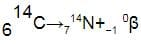Test Description

## 5 Questions MCQ Test Physics Class 12 | Test: Nuclei: Radioactivity

Test: Nuclei: Radioactivity for NEET 2023 is part of Physics Class 12 preparation. The Test: Nuclei: Radioactivity questions and answers have been prepared according to the NEET exam syllabus.The Test: Nuclei: Radioactivity MCQs are made for NEET 2023 Exam. Find important definitions, questions, notes, meanings, examples, exercises, MCQs and online tests for Test: Nuclei: Radioactivity below.
Solutions of Test: Nuclei: Radioactivity questions in English are available as part of our Physics Class 12 for NEET & Test: Nuclei: Radioactivity solutions in Hindi for Physics Class 12 course. Download more important topics, notes, lectures and mock test series for NEET Exam by signing up for free. Attempt Test: Nuclei: Radioactivity | 5 questions in 10 minutes | Mock test for NEET preparation | Free important questions MCQ to study Physics Class 12 for NEET Exam | Download free PDF with solutions
 1 Crore+ students have signed up on EduRev. Have you?
Test: Nuclei: Radioactivity - Question 1

### The control rods in a nuclear reactor

Detailed Solution for Test: Nuclei: Radioactivity - Question 1

Control rods are used in nuclear reactors to control the fission rate of uranium and plutonium. They are composed of chemical elements such as boron, silver, indium and cadmium that are capable of absorbing many neutrons without themselves fissioning.

Test: Nuclei: Radioactivity - Question 2

### A sample of radioactive material contains 1018 atoms. The half life of the material is 2 days, then the activity of the sample is​

Detailed Solution for Test: Nuclei: Radioactivity - Question 2

To find activity of the sample --->which is the rate of disintegration.

Since radioactivity comes under 1o kinetics.

[R]=k[A]     [A]-->amount of initial sample 1018 atoms

Given,

Half-life=2days

K=0.693/2x24x60x60 sec

R=(0.693/2x24x60x60)x1018

R≈3.5x1012 Bq

Test: Nuclei: Radioactivity - Question 3

### γ (Gamma) rays are​

Detailed Solution for Test: Nuclei: Radioactivity - Question 3

A gamma ray, or gamma radiation, is a penetrating form of electromagnetic radiation arising from the radioactive decay of atomic nuclei. It consists of the shortest wavelength electromagnetic waves and so imparts the highest photon energy.

Test: Nuclei: Radioactivity - Question 4

When a radioactive nucleus emits a β – particle, the mass number of the atom

Detailed Solution for Test: Nuclei: Radioactivity - Question 4

A beta particle forms when a neutron changes the mass number stays the same.
Example
Carbon-14 decays into nitrogen-14 by emitting a beta particle. This is the balanced equation for the reaction:Notice that:

• the mass number of the nucleus stays the same, 14
• the atomic number of the nucleus increases by 1, from 6 to 7
• the numbers at the top and bottom give the same totals on both sides, as (7 - 1) = 6

Nitrogen nuclei have 7 protons, so their nuclear charge is +7. They have one more proton than carbon nuclei, which have 6 protons and so a nuclear charge of +6.

Test: Nuclei: Radioactivity - Question 5

The purpose of moderator in a nuclear reactor is​

Detailed Solution for Test: Nuclei: Radioactivity - Question 5

In nuclear engineering, a neutron moderator is a medium that reduces the speed of fast neutrons, ideally without capturing any, leaving them as thermal neutrons with only minimal (thermal) kinetic energy.

## Physics Class 12

157 videos|452 docs|213 tests
 Use Code STAYHOME200 and get INR 200 additional OFF Use Coupon Code